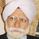8128瀏覽
When apply a strategy to Heikin Ashi Candle chart (HA candle), the strategy will use the open/close/high/low values of the Heikin Ashi candle to calculate the Profit and Loss, hence also affecting the Percent Profitable, Profit Factor, etc., often resulting a unrealistic high Percent Profitable and Profit Factor, which is misleading. But if you want to use the HA candle's values to calculate your indicator / strategy, but pass the normal candle's open/close/high/low values to the strategy to calculate the Profit / Loss, you can do this:

1) set up the main chart to be a normal candle chart
2) use this indicator script to plot a secondary window with indicator looks exactly like a HA-chart
3) to use the HA-candle's open/close/high/low value to calculate whatever indicator you want (you may need to create a separate script if you want to plot this indicator in a separate indicator window)

```//@version=2
// Note:
//   if you only want to see the Heikin Ashi Candle but not the normal Candle,
//   change the overlay option to overlay=true, then hide the normal Candle
study("I_Heikin Ashi Candle", shorttitle="I_HA Candle", overlay=false)

// --------------- Calculating HA Candle's values
//  -- you can use either one of the methods below, they give the same values

//   Method 1 - calculate the HA candle's value by formula
haclose = (open + high + low + close) / 4
haopen = na(haopen) ? (open + close) / 2 : (haopen + haclose) / 2
hahigh = max(high, max(haopen, haclose))
halow = min(low, min(haopen, haclose))

//   Method 2 - calculate the HA candle's value by pine script function heikinashi()
// haclose = security(heikinashi(tickerid), period, close)
// haopen = security(heikinashi(tickerid), period, open)
// hahigh = security(heikinashi(tickerid), period, high)
// halow = security(heikinashi(tickerid), period, low)

// --------------- Using HA Candle's values to define indicators

// then use the haclose, haopen, hahigh, halow to calculate whatever indicators you want:
// e.g.

// 1. stochastic
// k = sma(stoch(haclose, hahigh, halow, 14), 3)
// d = sma(k, 3)

// 2. sma
// sma14 = sma(haclose, 14)

// 3. ema
// ema14 = ema(haclose, 14)

// --------------- Plotting
plotcandle(haopen, hahigh, halow, haclose, title='Heikin-Ashi', color=(haopen < haclose) ? green : red, wickcolor=gray)
// END```

## 評論It's strange because HA plotted by TV do not match HA plotted by your script, I took a screenshot but cannot attach it.

But I think it may be a problem with TV plotting... I wrote and rewrote a script several times checking multiple sources but the data never matches exactly what's displayed by TV.ildarius
@ildarius, I haven't worked on the script for a long time so I have forgotten a lot about its features. However, without checking the details, my first thought is that in your attached file (not a full screenshot) I guess your main chart is already a HA chart, and the secondary chart is generated by my script (I guess?), so it becomes a HA chart of a HA chart, which of course look different and it is not what you want.

To compare them you need to open two chart window, one using TV HA chart and one using a normal chart and generating the HA chart using the script. Hope it makes sense.For example I like to put ema on Heikin Ashi chart with HA data
But in buy sell signals i like to use orignal candlestic data can you suggest me any idea